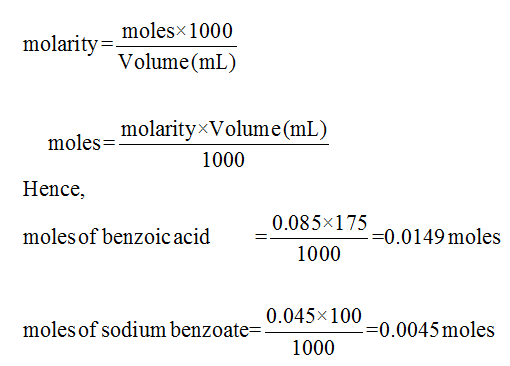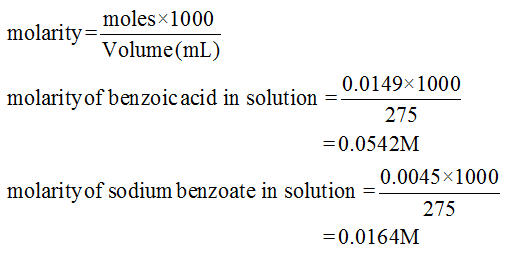# Determine the pH if a solution that consists of 175.0mL of 0.085M Benzoic acid and 100.0mL of 0.045M Sodium benzoate Ka of benzoic acid if 6.3x10-5

Question
1 views

Determine the pH if a solution that consists of 175.0mL of 0.085M Benzoic acid and 100.0mL of 0.045M Sodium benzoate

Ka of benzoic acid if 6.3x10-5

check_circle

Step 1

Given information:

Ka of benzoic acid = 6.3 × 10-5.

Benzoic acid = 175 mL of 0.085 M

Sodium benzoate = 100 mL 0.045 M

Thus,

Total volume of solution= 175 + 100= 275 mL

Step 2

Since,Step 3

As we know:...

### Want to see the full answer?

See Solution

#### Want to see this answer and more?

Solutions are written by subject experts who are available 24/7. Questions are typically answered within 1 hour.*

See Solution
*Response times may vary by subject and question.
Tagged in